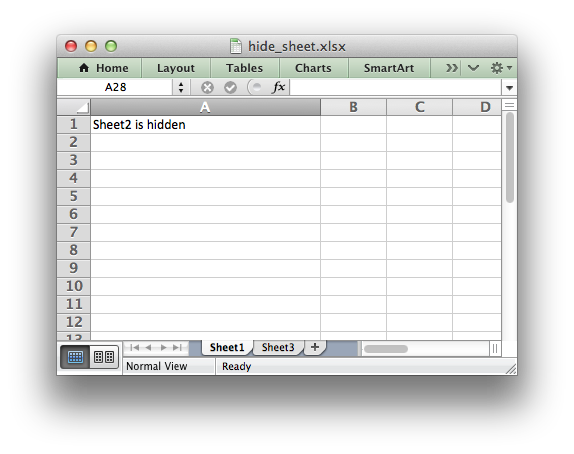# Example: Hiding Worksheets

This program is an example of how to hide a worksheet using the `hide()` method.```#######################################################################
#
# Example of how to hide a worksheet with XlsxWriter.
#
# Copyright 2013-2023, John McNamara, jmcnamara@cpan.org
#
import xlsxwriter

workbook = xlsxwriter.Workbook("hide_sheet.xlsx")

worksheet1.set_column("A:A", 30)
worksheet2.set_column("A:A", 30)
worksheet3.set_column("A:A", 30)

# Hide Sheet2. It won't be visible until it is unhidden in Excel.
worksheet2.hide()

worksheet1.write("A1", "Sheet2 is hidden")
worksheet2.write("A1", "Now it's my turn to find you!")
worksheet3.write("A1", "Sheet2 is hidden")

# Note, you can't hide the "active" worksheet, which generally is the
# first worksheet, since this would cause an Excel error. So, in order to hide
# the first sheet you will need to activate another worksheet:
#
#    worksheet2.activate()
#    worksheet1.hide()

workbook.close()
```